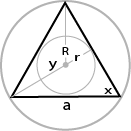## Ultimate Regular Polygons Calculator

Choose a regular polygon:
Choose a calculation:
 a = Length Units: Angle Units: Degrees Radians n = 3 r = 0 R = 0 A = 0 P = 0 x = 0 y = 0n = number of sides, a = side lengths, R = circumradius

r = inradius (apothem), P = perimeter, A = area, y = exterior angle, x = interior angle

» Show Work
Show steps.

The polygon calculator to calculate properties of a regular polygon. When you enter any 1 variable of the polygon like side lengths and selet the Number of Sides. It can Calculates side length, inradius (apothem), circumradius, area and perimeter and so on.

For example, To polygon calculator, side lengths is 3, number of sides is 6:

1. To calculate r when given n and a, use the following formula: (1/2)a * cot(π/n). When you substitute n and a for their values, you get (1/2)3 * cot(π/3), which equals 0.86603 m.
2. To calculate R when given n and a, use the following formula: (1/2)a * cot(π/n). When you substitute n and a for their values, you get (1/2)3 * csc(π/3), which equals 1.73205 m.
3. To calculate A when given n and a, use the following formula: (1/4)na2 * cot(π/n). When you substitute n and a for their values, you get (1/4)*3*32 * cot(π/3), which equals 3.89711 m2.
4. To calculate P when given n and a, use the following formula: na. When you substitute n and a for their values, you get 3 * 3, which equals 9 m.
5. To calculate x in degrees, use the following formula: (((n-2)/n) * 180). When you substitute n for its value, you get (((3-2)/3) * 180, which equals 60°.
6. To calculate y in degrees, use the following formula: 360 / n. When you substitute n for its value, you get 360 / 3, which equals 120°.
Thinkcalculator.com provides you helpful and handy calculator resources.